Hello, readers welcome to the new tutorial. In this post, we will learn How Data Flow in LabView. LABVIEW is the software that does not lie in another category of programing language. In this tool instead of using the text-based program, there is the graphical representation is called G is used to form the function. Data flow is used to see the flow of data during project implementation. Also named as streaming procedure.

Dataflow in this tool helps us to see the basic main execution of the program from right to left or top to bottom. In this post, we will construct a practical project and learn how data flow occurs in the LABVIEW. So let get started.

## How Data Flow in LabView

• For a detailed understanding of the data flow, we will construct a temperature conversion program in which Fahrenheit will convert to centigrade.
• To make a convertion the Fahrenheit to centigrade move to the front panel and press right then numeric and select the thermometer as shown in below figure.• When we place temperature in the front panel their resultant will be shown in the block diagram. Here you can see I have selected two temperatures sliders first for Fahrenheit and the other for centigrade.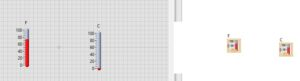• The formula for Fahrenheit to centigrade is given here

C=(F−32)×5/9

• Now we will apply it to make the function and will see the data flow process.
• Move to block diagram and press right and then numeric and select the multiply, subtract and division tabs for making circuits can see here.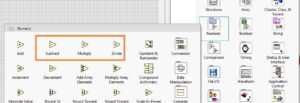• Also you can this component in the block diagram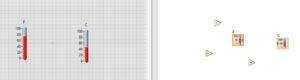• Now link all components and make projects as can see here
• Our final project will look like this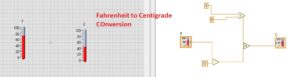• Now we will see how data flow occurs
• For a view of data, flow move to top bar of the block diagram and press at the highlight execution button.
• After that run your program and in the below figure you can see the practical data flow.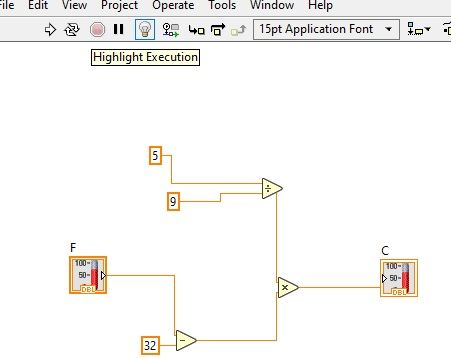• Here you can see that data is flowing between different objects and nodes when we use the highlight execution button then run our program.• For further understanding, you can see a different program like the addition of two numbers and apply the data execution tab to observe the practical working

That is all about the How Data Flow in LabView. I have explained all parameters to understand for data flow. If still have any further queries mention in the below comments. Thanks for reading have a nice day